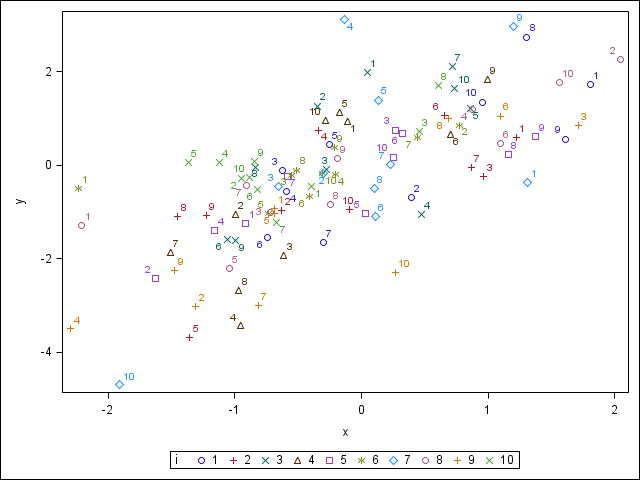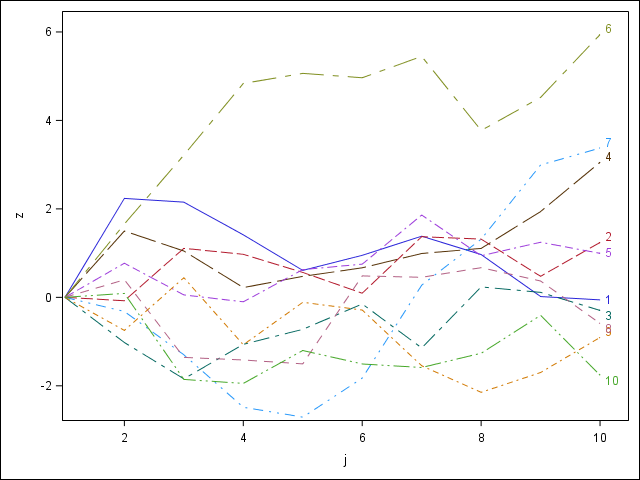## Labels in SGPLOT

In SGPLOT, DATALABEL or CURVELABEL can be used to write some contents inside.I have two questions.

1. When SGPLOT SCATTER, can I completely alter the symbols (dots, triangles, etc.) by their DATALABEL? In the attached example, I want to delete the symbols but want to alter them by the numbers.

2. When SGPLOT SERIES, can I automatically put the very ending numbers of the groups as CURVELABEL? CURVELABEL puts the group names, but I need the last values of the lines.

Here I append the code.

``````data _;
do i=1 to 10;
do j=1 to 10;
x=rannor(1);
y=x+rannor(1);
if j=1 then z=0;
else z+rannor(1);
output;
end;
end;
run;
ods listing gpath="!userprofile\desktop\";
ods graphics/reset=index;
ods results=off;
proc sgplot;
scatter x=x y=y/group=i datalabel=j;
run;
proc sgplot;
series x=j y=z/group=i curvelabel;
run;
ods results=on;
quit;``````

1 ACCEPTED SOLUTION

Accepted Solutions

## Re: Labels in SGPLOT

2. Yes. But you need to modify your data a little bit. You can specify a variable as the label, depending on the version of SAS, assuming the newest, so leave the variable empty for every other value except the Last values and those will be displayed.

AFAIK you can’t do #1 but I’d wait to see what others have to say.

@Junyong wrote:

In SGPLOT, DATALABEL or CURVELABEL can be used to write some contents inside.I have two questions.

1. When SGPLOT SCATTER, can I completely alter the symbols (dots, triangles, etc.) by their DATALABEL? In the attached example, I want to delete the symbols but want to alter them by the numbers.

2. When SGPLOT SERIES, can I automatically put the very ending numbers of the groups as CURVELABEL? CURVELABEL puts the group names, but I need the last values of the lines.

Here I append the code.

``````data _;
do i=1 to 10;
do j=1 to 10;
x=rannor(1);
y=x+rannor(1);
if j=1 then z=0;
else z+rannor(1);
output;
end;
end;
run;
ods listing gpath="!userprofile\desktop\";
ods graphics/reset=index;
ods results=off;
proc sgplot;
scatter x=x y=y/group=i datalabel=j;
run;
proc sgplot;
series x=j y=z/group=i curvelabel;
run;
ods results=on;
quit;``````

4 REPLIES 4

## Re: Labels in SGPLOT

2. Yes. But you need to modify your data a little bit. You can specify a variable as the label, depending on the version of SAS, assuming the newest, so leave the variable empty for every other value except the Last values and those will be displayed.

AFAIK you can’t do #1 but I’d wait to see what others have to say.

@Junyong wrote:

In SGPLOT, DATALABEL or CURVELABEL can be used to write some contents inside.I have two questions.

1. When SGPLOT SCATTER, can I completely alter the symbols (dots, triangles, etc.) by their DATALABEL? In the attached example, I want to delete the symbols but want to alter them by the numbers.

2. When SGPLOT SERIES, can I automatically put the very ending numbers of the groups as CURVELABEL? CURVELABEL puts the group names, but I need the last values of the lines.

Here I append the code.

``````data _;
do i=1 to 10;
do j=1 to 10;
x=rannor(1);
y=x+rannor(1);
if j=1 then z=0;
else z+rannor(1);
output;
end;
end;
run;
ods listing gpath="!userprofile\desktop\";
ods graphics/reset=index;
ods results=off;
proc sgplot;
scatter x=x y=y/group=i datalabel=j;
run;
proc sgplot;
series x=j y=z/group=i curvelabel;
run;
ods results=on;
quit;``````

## Re: Labels in SGPLOT

For #1 you can use a data attribute map. I'd recommend changing colours for you I variable and using different shapes/fills for your j values.

Data attribute maps are the way to do this https://documentation.sas.com/?docsetId=grstatproc&docsetTarget=n18szqcwir8q2nn10od9hhdh2ksj.htm&doc...DanH_sas
SAS Super FREQ

## Re: Labels in SGPLOT

If I interpret your request for #1 correctly, I think you should use a TEXT plot instead of a SCATTER plot. Use the variable from the SCATTER DATALABEL as both the TEXT variable and the GROUP variable, something like the following:

``text x=x y=y text=j / group=j;``

@Reeza's suggestion for #2 is the best solution, and should work for any version of SAS supporting ODS Graphics.

Hope this helps!

Dan

## Re: Labels in SGPLOT

@Junyong wrote:

In SGPLOT, DATALABEL or CURVELABEL can be used to write some contents inside.I have two questions.

1. When SGPLOT SCATTER, can I completely alter the symbols (dots, triangles, etc.) by their DATALABEL? In the attached example, I want to delete the symbols but want to alter them by the numbers.

I am not sure exactly what you mean by "delete the symbols but want to alter them by the numbers". If you really mean that you only want to display the number value an no marker at all them perhaps you want a text plot:

```proc sgplot ;
text x=x y=y text=i /group=i;
run;
```
Discussion stats
• 4 replies
• 3198 views
• 4 likes
• 4 in conversation